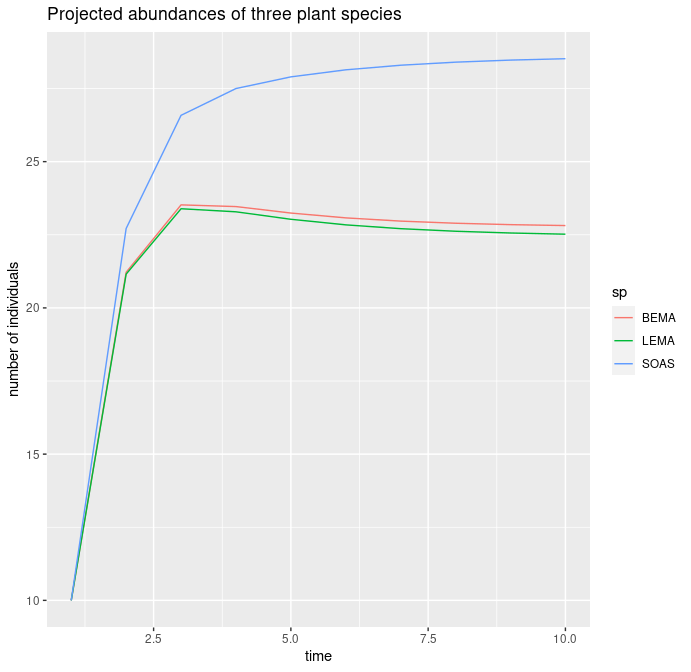# Projecting species abundances

Projecting species abundances

Population dynamics models, like the ones included in cxr, are used for understanding the influence of different factors in shaping species densities, and also for inferring future dynamics based on the expected factors. We have included in cxr basic functionality for projecting these dynamics. For that, we can use any of the default models included in the package, or -again- implement any user-defined model.

In this vignette, we show how to project the abundances of three species from our dataset a number of timesteps. For that, we first estimate model coefficients with the cxr_pm_multifit function, as in previous vignettes.

library(cxr)
data("neigh_list")

three_sp <- c("BEMA","LEMA","SOAS")
sp.pos <- which(names(neigh_list) %in% three_sp)

data <- neigh_list[sp.pos]
# keep only fitness and neighbours columns
for(i in 1:length(data)){
data[[i]] <- data[[i]][,2:length(data[[i]])]
}
focal_column <- names(data)

# Beverton-Holt model
model_family <- "BH"

# the "bobyqa" algorithm works best for these species
optimization_method <- "bobyqa"

# pairwise alphas, but no covariate effects
alpha_form <- "pairwise"
lambda_cov_form <- "none"
alpha_cov_form <- "none"

# no fixed terms, i.e. we fit both lambdas and alphas
fixed_terms <- NULL

# for demonstration purposes
bootstrap_samples <- 3

# a limited number of timesteps.
timesteps <- 10

# for demonstration purposes
initial_abundances <- c(10,10,10)
names(initial_abundances) <- three_sp

# standard initial values,
# not allowing for intraspecific facilitation

initial_values <- list(lambda = 10,
alpha_intra = 0.1,
alpha_inter = 0.1)
# lambda_cov = 0.1,
# alpha_cov = 0.1)
lower_bounds <- list(lambda = 1,
alpha_intra = 0,
alpha_inter = -1)
# lambda_cov = 0,
# alpha_cov = 0)
upper_bounds <- list(lambda = 100,
alpha_intra = 1,
alpha_inter = 1)
# lambda_cov = 1,
# alpha_cov = 1)

With all initial values set, we can fit the parameters.

  cxr_fit <- cxr_pm_multifit(data = data,
focal_column = focal_column,
model_family = model_family,
# covariates = salinity,
optimization_method = optimization_method,
alpha_form = alpha_form,
lambda_cov_form = lambda_cov_form,
alpha_cov_form = alpha_cov_form,
initial_values = initial_values,
lower_bounds = lower_bounds,
upper_bounds = upper_bounds,
fixed_terms = fixed_terms,
bootstrap_samples = bootstrap_samples)

Projecting abundances from a cxr object is straightforward: just call the function abundance_projection with the cxr fit and the number of timesteps to project as well as the initial abundances. In this example, our fit did not include the effect of covariates, but if this was the case, we would also need to specify the value of each covariate in the projected timesteps (see the help of abundance_projection for details).

  ab <- abundance_projection(cxr_fit = cxr_fit,
# covariates = covariates_proj,
timesteps = timesteps,
initial_abundances = initial_abundances)

This function returns a simple numeric matrix with the projected abundances. Lastly, here is a basic plot showing the projection. This is a pedagogical example and it does not make sense to interpret it ecologically, but it shows how one of the three species selected tends to become more abundant in the short term.

ab.df <- as.data.frame(ab)
ab.df\$timestep <- 1:nrow(ab.df)
ab.df <- tidyr::gather(ab.df,key = "sp",value = "abund",-timestep)
abund.plot <- ggplot2::ggplot(ab.df,
ggplot2::aes(x = timestep,
y = abund, group = sp)) +
ggplot2::geom_line(ggplot2::aes(color = sp)) +
ggplot2::ylab("number of individuals") + ggplot2::xlab("time") +
ggplot2::ggtitle("Projected abundances of three plant species")+
NULL
abund.plotAs with other features of cxr, you can implement your own model for projecting species abundances. If you have gone through vignette 4, you should already be familiar with the format of cxr models, and how to make them available to the package.

Here, for reference, we show the complete code of the most complex Beverton-Holt model included. You can use this example as a template for the function name and arguments. The instructions of vignette 4 are also applicable here.

#' Beverton-Holt model for projecting abundances,
#' with specific alpha values and global covariate effects on alpha and lambda
#'
#' @param lambda named numeric lambda value.
#' @param alpha_intra single numeric value.
#' @param alpha_inter numeric vector with interspecific alpha values.
#' @param lambda_cov numeric vector with effects of covariates over lambda.
#' @param alpha_cov named list of named numeric vectors
#' with effects of each covariate over alpha values.
#' @param abundance named numeric vector of abundances in the previous timestep.
#' @param covariates matrix with observations in rows and covariates in named columns.
#' Each cell is the value of a covariate in a given observation.
#'
#' @return numeric abundance projected one timestep
#' @export
BH_project_alpha_pairwise_lambdacov_global_alphacov_pairwise <- function(lambda,
alpha_intra,
alpha_inter,
lambda_cov,
alpha_cov,
abundance,
covariates){

# put together intra and inter coefficients,
# be sure names match

spnames <- names(abundance)

alpha <- c(alpha_intra,alpha_inter)
alpha <- alpha[spnames]
alpha_covs <- list()
for(ia in 1:length(alpha_cov)){
alpha_covs[[ia]] <- alpha_cov[[ia]][spnames]
}

numsp <- length(abundance)
expected_abund <- NA_real_

# model
num = 1
focal.cov.matrix <- as.matrix(covariates)
for(v in 1:ncol(focal.cov.matrix)){
num <- num + lambda_cov[v]*focal.cov.matrix[,v]
}
cov_term_x <- list()
for(v in 1:ncol(focal.cov.matrix)){
cov_temp <- focal.cov.matrix[,v]
for(z in 1:length(abundance)){
#create  alpha_cov_i*cov_i vector
cov_term_x[[z+(length(abundance)*(v-1))]] <-
# alpha_cov[z+(ncol(abund)*(v-1))]
alpha_cov[[v]][z] * cov_temp
}
}
cov_term <- list()
for(z in 0:(length(abundance)-1)){
cov_term_x_sum <- cov_term_x[[z+1]]
if(ncol(focal.cov.matrix) > 1){
for(v in 2:ncol(focal.cov.matrix)){
cov_term_x_sum <- cov_term_x_sum +
cov_term_x[[v + length(abundance)]]
}
}
cov_term[[z+1]] <- cov_term_x_sum
}
term <- 1 #create the denominator term for the model
for(z in 1:length(abundance)){
term <- term + (alpha[z] + cov_term[[z]]) * abundance[z]
}
expected_abund <- (lambda * (num) / term) * abundance[names(lambda)]
expected_abund
}